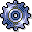Target Cost CalculationUse

To achieve useful comparisons of the plan and actual costs of a period, you need a consistent basis. Plan and actual costs are compared on the basis of actual activity.

The system uses the operating rate to adjust the plan costs of a period to the actual activity.

The operating rate is based on the following formula:

Operating rate = (Actual activity ¸ Plan activity) X 100

The plan costs adapted to actual costs in this manner are called target costs. In variance calculation, the system calculates target costs for each cost center and business process in each period. The values are calculated according to the following formulas:

• For activity-dependent costs that were not incurred through internal activity allocations:
The value-based viewpoint is in the foreground here, even if you enter additional quantities during planning.

Fixed target quantity = Fixed plan quantity

Variable target quantity = Variable plan quantity X Operating rate

Fixed target costs = Fixed plan costs

Variable target costs = Variable plan costs X Operating rate

Total target costs = Fixed plan costs + Variable plan costs X Operating rate

For these costs, the emphasis is on value-based calculation, even if additional quantities are entered when planning.

• For credit records from internal activity allocations:

Fixed target costs = Fixed plan costs X Operating rate

Variable target costs = Variable plan costs X Operating rate

If an activity type or a business process participate as senders in predistribution of fixed costs, the fixed costs are not corrected with the operating rate (see: Predistribution of Fixed Costs). In this case:

Fixed target costs = Fixed plan costs

• For all activity-independent costs:

Fixed target costs = Fixed plan costs

Variable target costs = Variable plan costs

• For activity-dependent costs from internal activity allocation.

Fixed target quantity = Fixed plan quantity

Variable target quantity = Variable plan quantity X Operating rate

Fixed target costs = Fixed target quantity X Fixed price

+ Fixed target quantity X Variable price

+ Variable target quantity X Fixed price

Variable target costs = Variable target quantity X Variable price.

Here, the emphasis is on quantity-based calculation because allocation takes place via activity quantities. The target costs can vary with the operating rate because the variable target quantity is included in the fixed target costs.

If during activity allocation you assigned the fixed costs using predistribution of fixed costs, (see: Predistribution of Fixed Costs), the target costs are calculated as follows:

Fixed target costs = Fixed plan costs

Variable target costs = Variable plan costs X Operating rate

In this case, the fixed costs are not dependent on the operating rate.